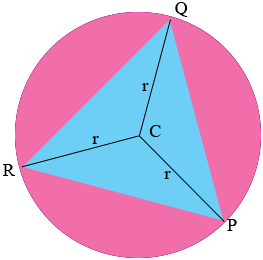SEARCH HOMEMath Central Quandaries & QueriesQuestion from Olyana, a student: I am struggling with this question! Help! So, there is a circle. In the circle, there is an equilateral triangle inscribed. Each side of the triangle is 20. There is no other info given, other than the triangle is inscribed in the circle and the sides of the triangle are 20. I am supposed to find the radius of the circle! Please help!Hi Olyana,$C$ is the centre of the circle and $r$ is its radius. What do you know about the triangle $PQC?$

Write back if you need more assistance,
PennyMath Central is supported by the University of Regina and the Imperial Oil Foundation.